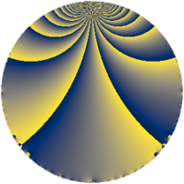# Properties

 Label 60.2.jLevel $60$ Weight $2$ Character orbit 60.j Rep. character $\chi_{60}(7,\cdot)$ Character field $\Q(\zeta_{4})$ Dimension $12$ Newform subspaces $1$ Sturm bound $24$ Trace bound $0$

# Related objects

## Defining parameters

 Level: $$N$$ $$=$$ $$60 = 2^{2} \cdot 3 \cdot 5$$ Weight: $$k$$ $$=$$ $$2$$ Character orbit: $$[\chi]$$ $$=$$ 60.j (of order $$4$$ and degree $$2$$) Character conductor: $$\operatorname{cond}(\chi)$$ $$=$$ $$20$$ Character field: $$\Q(i)$$ Newform subspaces: $$1$$ Sturm bound: $$24$$ Trace bound: $$0$$

## Dimensions

The following table gives the dimensions of various subspaces of $$M_{2}(60, [\chi])$$.

Total New Old
Modular forms 32 12 20
Cusp forms 16 12 4
Eisenstein series 16 0 16

## Trace form

 $$12 q - 4 q^{6} - 12 q^{8} + O(q^{10})$$ $$12 q - 4 q^{6} - 12 q^{8} - 8 q^{10} - 8 q^{12} - 4 q^{13} + 12 q^{16} - 20 q^{17} + 20 q^{20} + 12 q^{22} - 20 q^{25} + 16 q^{26} - 4 q^{28} + 8 q^{30} + 20 q^{32} + 8 q^{33} + 4 q^{36} + 4 q^{37} + 16 q^{38} - 8 q^{40} + 16 q^{41} + 20 q^{42} + 4 q^{45} - 40 q^{46} + 16 q^{48} - 16 q^{50} - 8 q^{52} + 4 q^{53} - 64 q^{56} - 20 q^{58} - 20 q^{60} - 32 q^{61} - 56 q^{62} + 20 q^{65} - 24 q^{66} - 16 q^{68} + 44 q^{70} - 12 q^{72} + 44 q^{73} + 8 q^{76} + 48 q^{77} - 24 q^{78} + 4 q^{80} - 12 q^{81} + 16 q^{82} + 44 q^{85} + 64 q^{86} + 60 q^{88} + 12 q^{90} + 56 q^{92} - 16 q^{93} + 44 q^{96} - 20 q^{97} + 24 q^{98} + O(q^{100})$$

## Decomposition of $$S_{2}^{\mathrm{new}}(60, [\chi])$$ into newform subspaces

Label Dim $A$ Field CM Traces $q$-expansion
$a_{2}$ $a_{3}$ $a_{5}$ $a_{7}$
60.2.j.a $12$ $0.479$ 12.0.$$\cdots$$.1 None $$0$$ $$0$$ $$0$$ $$0$$ $$q-\beta _{6}q^{2}-\beta _{2}q^{3}+(\beta _{2}+\beta _{4}-\beta _{7})q^{4}+\cdots$$

## Decomposition of $$S_{2}^{\mathrm{old}}(60, [\chi])$$ into lower level spaces

$$S_{2}^{\mathrm{old}}(60, [\chi]) \cong$$ $$S_{2}^{\mathrm{new}}(20, [\chi])$$$$^{\oplus 2}$$